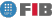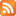# Numerical Computation (CNU)

Credits Dept. Type Requirements
7.5 (6.0 ECTS) MAT
• Elective for DIE
• Elective for DCSFW
• Elective for DCSYS
AL - Prerequisite for DIE , DCSYS , DCSFW
CAL - Prerequisite for DIE , DCSYS , DCSFW

## Instructors

 Person in charge: (-) Others: (-)

## General goals

This subject offers an extensive overview of numerical analysis in order for students to gain a good understanding of both fundamental topics and for them to familiarise themselves with the concepts, basic methods, current techniques, applications for PCs and current libraries in the working world. The first and second parts of the subject introduce more basic, fundamental material, while the third part places a greater emphasis on solving the sorts of equations that all engineers must understand and be able to apply: equations with derivatives in which a sufficiently close first approach to the subject matter means that students will come away with the concepts and tools they need to be able to interpret the results. The subject focuses on opening students' minds to as wide a range of methods and applications as possible, so that they end up with a solid background as programmers and users of numerical methods.

## Specific goals

### Knowledges

1. Analysis, programming, interpretation and verification of results, prediction and documentation of a mathematical model.
Ability to recognise epsilon on the computer one is working with.
Calculation of numerical functions, propagation error, and data representation.
Ability to study a problem and its numerical stability: poorly-conditioned problems.
Effective calculation in series and the scope for accelerating convergence.
2. Learn to differentiate between interpolation and approximation to functions.
Master interpolation methods: linear system, Lagrange, Newton, and Tchebichev. Learn the advantages and disadvantages of each method.
Differentiate between Lagrange interpolating polynomials and Hermite interpolation, and learn which one to use depending on the case.
Choose an approximation method: error in choice of nodes; minimum quadratic error; sub-infinite rule error in an interval.
3. Evaluate whether to use a direct or iterative solution depending on the size of the system. Estimate the condition number of a system matrix.
Calculate the impact of the values and their application to various models.
Study of the most appropriate integration methods in terms of precision and time, depending on the function and integration interval.
Extrapolate from a given formula. Repeated extrapolation. Application to the calculation of integrals.
Construct an adaptive integration formula, given a formula.
Evaluation of Gaussian integration.
4. Analyze and decide the most efficient method for calculating a non-linear equation. Study the concept of order and the computational cost of iterative methods.
5. Master numerical integration methods for the simplest differential equations. The problems involved in reducing the sise of the integration step or opting for too large a step when seeking shorter calculation times.
6. Understand function minimisation.
Learn how to incorporate a tolerance in the calculation, make allowance for the number of iterations required, introduce a set of initial approximations, and apply the problem to examples of varying difficulty.
Consider the possibilities presented by a problem, and achieve the greatest adaptability to such variations.
7. Discretise the partial derivatives, analyze local and global errors in the problem, and solve the associated linear system.
8. Learn how to incorporate a tolerance in the calculation, make allowance for the number of iterations required, introduce a set of initial approximations, and apply the problem to examples of varying difficulty.
9. Consider the possibilities presented by a problem, and achieve the greatest adaptability to such variations.

### Abilities

1. Programming in a scientific language for PCs - Matlab, Maple, Octave, Maxima - and for large computers -Fortran, C++-.
2. Use programme libraries for numerical calculations - free: Forsythe, Kahaner, Press, or more general libraries: IMSL, NAG-.
3. Writing scientific documents, using LATEX to incorporate mathematical formulae.
4. Ability to combine symbolic manipulators and numerical tools in order to exploit the contributions of each to the overall process.
Knowledge of maths that can immediately be applied to computers. Growth of a maths culture, with applications in various fields.
5. Willingness and ability to apply mathematics-based scientific method to problems.

Ability to form part of a multidisciplinary work team.

### Competences

1. Ability to create and use models of reality.
2. Ability to solve problems through the application of scientific and engineering methods.
3. Ability to design systems, components and processes meeting certain needs, using the most appropriate methods, techniques and tools in each case.
4. Ability to act independently: Know how to work on one"s own with just the bare minimum of knowledge and guidance.
5. Intellectual curiosity and openness.

## Contents

Estimated time (hours):

 T P L Alt Ext. L Stu A. time Theory Problems Laboratory Other activities External Laboratory Study Additional time

1. PRELIMINARIES
T      P      L      Alt    Ext. L Stu    A. time Total
3,0 3,0 2,0 0 3,0 6,0 0 17,0
Introduction to the course; Methodology; Programme; Bibliography; Evaluation.

What is CNU? Mathematical modelling. Sources of error, and the stability of algorithms.

Floating point arithmetical representation. Error analysis.

Calculating series. Accelerating convergence.

2. POLYNOMIAL INTERPOLATION
T      P      L      Alt    Ext. L Stu    A. time Total
3,0 3,0 1,0 0 1,0 6,0 0 14,0
Polynomial interpolation: Lagrange Method. Newton divided difference method.

Interpolation errors. Choice of nodes. Tchebichev polynomials.

Runge"s phenomenon. Hermite interpolation.

3. LINEAR SYSTEMS AND EIGEN VALUES
T      P      L      Alt    Ext. L Stu    A. time Total
7,0 4,0 1,0 0 2,0 9,0 0 23,0
Direct methods: Gaussian (Gauss-Jordan) elimination and LU factorisation.

Compact methods. Inverse calculations.

Introduction to matricial norms. Error constraints

Concept of eigen values and eigen vector. Associated real problems.

Iterative Methods: Jacobi and Gauss-Seidel methods. Convergence.

Powers and derivatives method.

Householder QR factorisation.

Eigen values for symmetric tridiagonal matrices. QR method.

4. NUMERICAL INTEGRATION
T      P      L      Alt    Ext. L Stu    A. time Total
5,0 3,0 1,0 0 2,0 5,0 0 16,0

Richardson"s Extrapolation.

Numerical integration: Newton-Côtes formulae. Romberg"s Method.

Gaussian integration.

5. ZEROS OF NON-LINEAR FUNCTIONS
T      P      L      Alt    Ext. L Stu    A. time Total
4,0 3,0 1,0 0 2,0 6,0 0 16,0

Nested interval methods and iterative methods.

Convergence order and method efficiency. Accelerating convergence.

6. INTRODUCTION TO ORDINARY DIFFERENTIAL EQUATIONS
T      P      L      Alt    Ext. L Stu    A. time Total
7,0 3,0 2,0 0 3,0 8,0 0 23,0
Initial value problems: Introductory examples. Pass methods. Multi-pass methods.

Differential equations. Consistency, stability, and convergence. Stiff equations.

Boundary value problems. The Finite Difference Method applied to linear problems.

7. INTRODUCTION TO PARTIAL DIFFERENTIAL EQUATIONS
T      P      L      Alt    Ext. L Stu    A. time Total
5,0 3,0 2,0 0 3,0 6,0 0 19,0
Introductory examples: heat and wave equations. Finite Difference Method and the Finite Elements Method.

Consistency, stability, and convergence. Numerical resolution.

 Total per kind T P L Alt Ext. L Stu A. time Total 34,0 22,0 10,0 0 16,0 46,0 0 128,0 Avaluation additional hours 10,0 Total work hours for student 138,0

## Docent Methodolgy

Theory classes: The theory classes will: present a real problem; define and construct concepts, methods and techniques needed to solve the problem; predict problems and indicate kindred situations.

Classes of problems: These classes will mainly be spent on solving problems that complement and/or extend the theory presented and examples thereof.

Lab classes: The lab classes will consist of study and visualisation of the algorithms covered in the theory class, using numerical software (Matlab, Octave, etc.) and symbolic manipulation tools (Maple, Maxima, etc). These exercises will be presented by the teacher in the PC classroom, and the students will continue work on them in interactive fashion in accordance with the prepared guidelines.

Practical assignments: each student has to carry out five short practical assignments in C++, corresponding to the first five chapters. These assignments will consist of an application for one or more routines set by the teacher. The aim is to solve a specific practical problem. Microsoft Developer Studio, which allows the use of Visual C, will be employed.

## Evaluation Methodgy

Overall grading of the assignments will be based on:

Lab classes: practical exercises with Matlab or Octave. The teacher will present the practical work for the lab classes. Students will then set to work on a series of prepared calculations. The work will not be submitted at the end of the session but rather by a set deadline (2 points);

Practical work in a classical programming language: Fortran or C in which the functions to be used have already been constructed, students having to write the main programme (2 points);

Two exams of problems, in which students may use calculators and books (2 + 2 points). The exams will be held in class hours (duration: two hours).

The final test is on fundamental theory (2 points). This consists of a series of questions requiring short answers.

## Basic Bibliography

• GRAU, Miquel; NOGUERA, Miquel: Càlcul numèric, Edicions UPC, 1993.
• Miquel Grau Sánchez, Miquel Noguera Batlle Cálculo numérico, Edicions UPC, 2001.
• Anton Aubanell, Antoni Benseny, Amadeu Delshams Eines bàsiques de càlcul numèric : amb 87 problemes resolts, Universitat Autònoma de Barcelona, 1991.
• J. Douglas Faires, Richard L. Burden Métodos numéricos, International Thomson Paraninfo, 2004.
• Richard L. Burden, J. Douglas Faires Análisis numérico, International Thomson, 2002.
• George E. Forsythe, Michael A. Malcolm, Cleve B. Moler Computer methods for mathematical computations, Prentice-Hall, 1977.
• Gene H. Golub, James M. Ortega Scientific computing and differential equations : an introduction to numerical methods, Academic Press, 1992.

## Complementary Bibliography

• Germund Dahlquist, Ake Björck Numerical methods, Dover,, 2003.
• Carl-Erik Fröberg Introducción al análisis numérico, Vicens-Vives, 1977.
• William H. Press ... [et al.] Numerical recipes. The art of scientific computing, Cambridge University Press, 2007.
• Anthony Ralston, Philip Rabinowitz A First course in numerical analysis, McGraw-Hill,, 1978.
• J.H. Wilkinson The Algebraic eigenvalue problem, Clarendon,, 1965.

1. http://www.math.ysu.edu/~faires/Numerical-Methods/

2. http://www.library.cornell.edu/nr/bookf90pdf.html

3. http://www.math.ysu.edu/~faires/Numerical-Analysis/

## Previous capacities

Students must have passed M1 and M2 courses during the selection stage.© Barcelona school of informatics - Contact -RSS
This website uses cookies to offer you the best experience and service. If you continue browsing, it is understood that you accept our cookies policy.
Classic version Mobile version